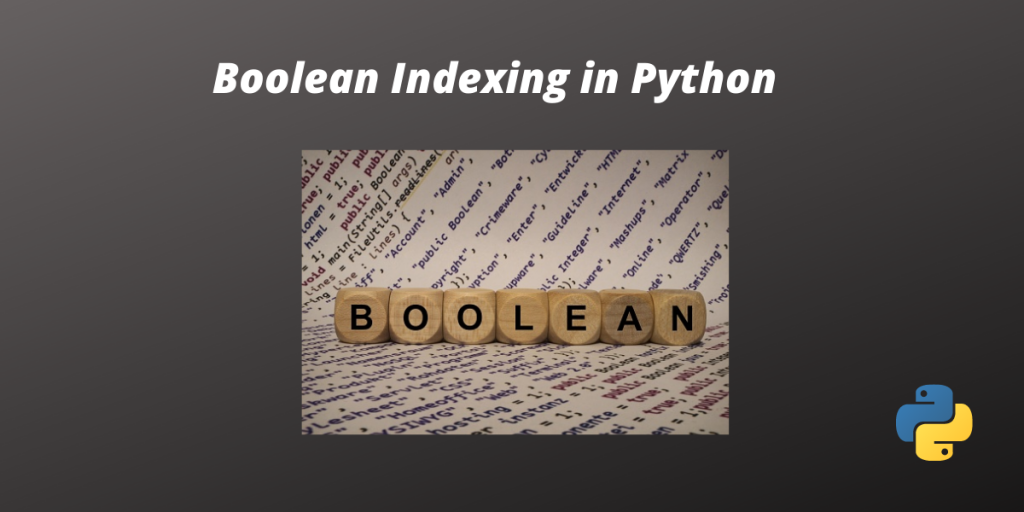# Boolean Indexing in Python – A Quick GuideIsn’t it interesting that Boolean values can be used as indexes in dataframes? Boolean indexes represent each row in a DataFrame. Boolean indexing can help us filter unnecessary data from a dataset. Filtering the data can get you some in-depth information that otherwise could not have been found. In this article, we will learn how to use Boolean indexing to filter and segment data. So let’s begin!

## Boolean Indexing in Python

Let’s start by creating a DataFrame. We will create a DataFrame using data on the age of a group of candidates taking part in a competition.

```import pandas as pd
# Creating a dictionary
data = {'Name':["Tommy","Linda","Justin","Brendon"], 'Age':[31,24,16,22]}
df = pd.DataFrame(data,index=[True,False,True,False])
print(df)
```

Output

```        Name         Age
True     Tommy   31
False    Linda   24
True    Justin   16
False  Brendon   22
```

### 1. Using the.loc [] function

This is an excellent and simple function that can help you filter your data according to the Boolean index. Using this function, we can filter out the data with a particular Boolean value. Let’s suppose we pass True to the .loc [] function, we will only get the filtered data having index values as True. We cannot use integers as Boolean values in this method.

For example:

```import pandas as pd
# Creating a dictionary
data = {'Name':["Tommy","Linda","Justin","Brendon"], 'Age':[31,24,16,22]}
df = pd.DataFrame(data,index=[True,False,True,False])
print(df.loc[True])
```

Output:

```        Name       Age
True   Tommy   31
True  Justin   16
```

### 2. Using .iloc[] function

iloc[] function only accepts integer values and so we need to pass integer values to the function.

For example:

```import pandas as pd
# Creating a dictionary
data = {'Name':["Tommy","Linda","Justin","Brendon"], 'Age':[31,24,16,22]}
df = pd.DataFrame(data,index=[1,0,0,1])
print(df.iloc)
```

Output:

```Name    Linda
Age        24
Name: 0, dtype: object

```

### 3. Using the .ix[] function

This is also a similar kind of method to the above one, but we can use integers as Boolean values in this case. So, for instance, if we assign the index values as 1 and 0, we can filter the rows having index values of 0 or 1.

```import pandas as pd
# Creating a dictionary
data = {'Name':["Tommy","Linda","Justin","Brendon"], 'Age':[31,24,16,22]}
df = pd.DataFrame(data,index=[1,1,0,0])
print(df.ix)
```

Output:

```           Name       Age
0       Justin          16
0       Brendon     22
```

## Conclusion

In summary, we learned how to use boolean indexing in python and filter the useful data. Hope you found this article helpful.